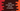# Kotlin program to find out the average marks of a list of students# Kotlin program to find out the average marks of a list of students :

In this Kotlin example program, we will learn how to find the average marks of a list of students. Our program will create one list of few students first and then it will find out the average marks of these students.

We will show you two different ways to find out the average. The first method will show you the traditional way by using a loop and the second method will use built-in average method.

This program will give you better understanding on how to use for-loops, iterating list and how to use data-class in kotlin.

## Method 1 : Using a for-loop :

Using a loop, we can iterate through a list and find out the sum of marks of the list of students. Then, we can divide it by the size of the list to find out the average value. Let’s take a look at the program :

``````//1
data class Student(val name: String, val marks: Int)

fun main(args: Array) {

//2
val studentList = listOf(Student("Alex", 40), Student("Brook", 32), Student("Carlos", 39), Student("Albert", 55), Student("Mark", 66), Student("Jack", 25), Student("Rose", 44), Student("Mary", 22))

//3
var totalMarks = 0

//4
for (student in studentList) {
totalMarks += student.marks
}

//5
val avgMarks = totalMarks / studentList.size

print("Average marks : %d\n".format(avgMarks))
}
``````

### Explanation :

The commented numbers in the above program denote the step number below :

1. Student is a data-class. These types of classes are used to create simple model class. This class can hold two different types of values : name of String type and marks of Int type.
2. In the main function, we are creating one list of 8 different students. We are using listOf method to create the list. Each Student has different name and marks.
3. Create one variable totalMarks with value 0.
4. Now iterate through the list elements one by one by using a for loop. Inside the loop, for each element of the loop, add its marks to the totalMarks variable. So, we are adding marks of all Student to the totalMarks variable.
5. Finally, calculate the average marks by dividing the total marks by the size of the list. And print out the result.

It will print the below output :

``````Average marks : 40
``````

## Method 2 : Using average() :

Instead of using a loop, we can also use map and average() to find out the average from a list only in one line. The complete program will look like as below :

``````data class Student(val name: String, val marks: Int)

fun main(args: Array) {

val studentList = listOf(Student("Alex", 40), Student("Brook", 32), Student("Carlos", 39), Student("Albert", 55), Student("Mark", 66), Student("Jack", 25), Student("Rose", 44), Student("Mary", 22))

val avgMarks = studentList.map { s -> s.marks }.average().toInt()

print("Average marks : %d\n".format(avgMarks))
}
``````

As you can see that the average value avgMarks is calculated only in one line instead of iterating it using a loop. The return value of average() is Double and that’s why we are using toInt() to convert it to an integer. The output of the program will be same as the previous one :

``````Average marks : 40
``````

### Conclusion :

In this tutorial, we have learn how to find the average marks of a list of students in Kotlin. Both of the methods explained above will result the same output but second one is more preferable in kotlin. Try to run the examples and drop one comment below if you have any queries.

You might also like :